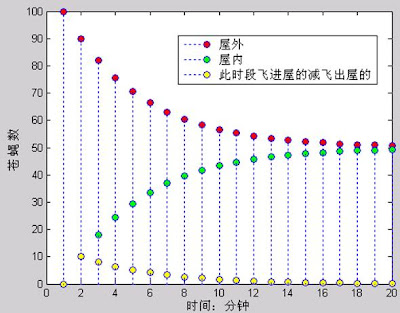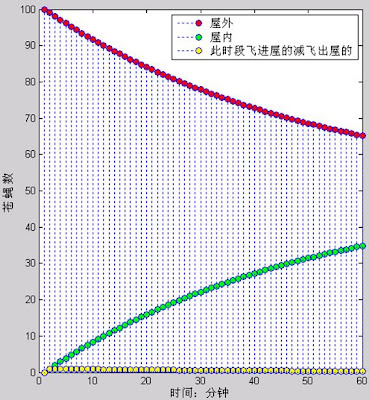我爱民科，苍蝇越多越干净？！

“怎么苍蝇老飞进家里，然后不飞出去啊，是不是家里太脏了？”

1. 苍蝇互相独立，自己决定自己怎么飞，也就是说不会发生“苍蝇女对苍蝇男说：我们一起去逛街吧 & 苍蝇男被迫同意的情况；

2. 苍蝇比较低等，所以把它们的飞行近似的鄙视为布朗运动，随机的撞到窗户，且简化的认为你家窗子面积上，认为每个点出现苍蝇的概率是一样的。

1. 单位时间T，是“每只苍蝇撞窗户时间间隔”的经验值，可以基于对某地形下大量苍蝇的统计获得；

2. 纱窗不小心留出的缝占整个窗户面积的比例为P = Areaopen/Areaall。，而苍蝇只要撞到缝所在地就会从屋外飞到屋内，反之亦然；

3. 矩阵：

FlyNum(t) = [ OutNum(t), InNum(t)]

OutNum(t+T) = OutNum(t)×(1-P) + InNum(t)×P;

InNum(t+T) = OutNum(t)×P + InNum(t)×(1-P);

FlyNum(t+T) = [ OutNum(t+T), InNum(t+T)];

Sum(FlyNum(t+T)) = OutNum(t+T) + InNum(t+T) = …

(OutNum(t)×(1-P) + InNum(t)×P) + (OutNum(t)×P + InNum(t)×(1-P)) = …

OutNum(t) + InNum(t) = Sum(FlyNum(t));

3. 实验的时间段内，室内外的苍蝇总数不能变，也就是说“屋内外”要是一个封闭系统。

FlyNum(0) = [ 100, 0]

FlyNum(T) = [ 90, 10]

FlyNum(2T) = [ 82, 18]

……------------------------------------------------------------如果你想第一时间看到最新内容, 就猛击此处订阅吧!

《我爱民科，苍蝇越多越干净？！》有4个想法

1. 熙雨说道：

这个链接点进去是攻击站点，不安全么？？

2. @熙雨
可能那个网站做得不好～ 非专业网站经常这样的

3. 熙雨说道：

@iamsujie
嗯，那不是没的看了啊

4. 李冰说道：

布朗运动的化。。。。必然是这个结果撒